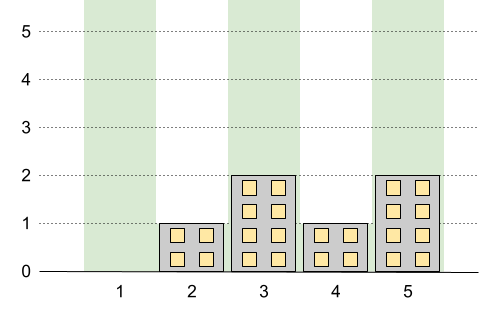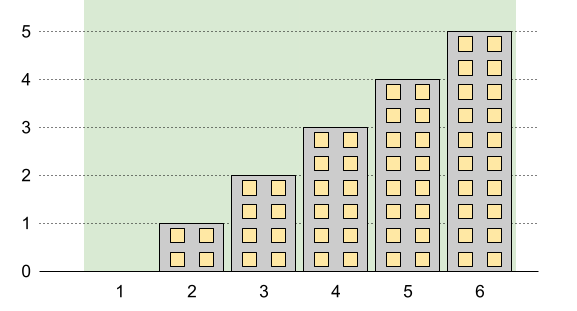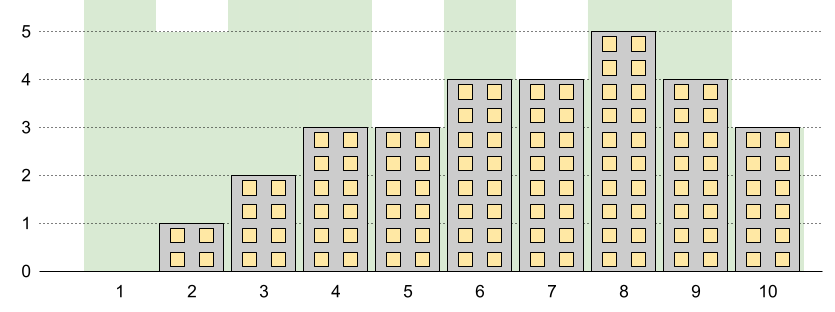# 最高建筑高度

## 1840. 最高建筑高度 (Hard)

• 每栋建筑的高度必须是一个非负整数。
• 第一栋建筑的高度 必须 是 `0` 。
• 任意两栋相邻建筑的高度差 不能超过  `1` 。```输入：n = 5, restrictions = [[2,1],[4,1]]```输入：n = 6, restrictions = []

``````输入：n = 10, restrictions = [[5,3],[2,5],[7,4],[10,3]]

```

• `2 <= n <= 109`
• `0 <= restrictions.length <= min(n - 1, 105)`
• `2 <= idi <= n`
• `idi` 是 唯一的 。
• `0 <= maxHeighti <= 109`

[数组] [数学]